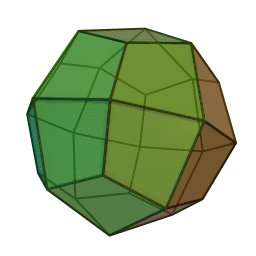# Wires With Will

A useless wire having a total resistance of $48 \space \Omega$ is cut into 48 equal pieces. Then, a regular Deltoidal Icositetrahedron as shown below.If the equivalent resistance between two opposite points, where four edges meet together is $R \space \Omega$, then enter your answer as the value of $100R$.

###### Image credit: Wikipedia
×

Problem Loading...

Note Loading...

Set Loading...